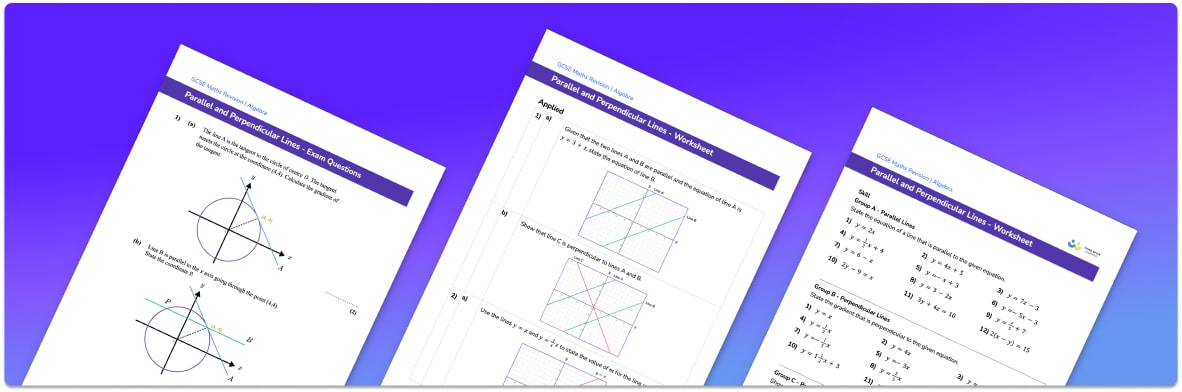# Parallel And Perpendicular Lines Worksheet• Section 1 of the parallel and perpendicular lines worksheet contains 20+ skills-based parallel and perpendicular lines questions, in 3 groups to support differentiation
• Section 2 contains 3 applied parallel and perpendicular lines questions with a mix of worded problems and deeper problem solving questions
• Section 3 contains 3 foundation and higher level GCSE exam style parallel and perpendicular lines questions
• Answers and a mark scheme for all parallel and perpendicular lines questions are provided
• Questions follow variation theory with plenty of opportunities for students to work independently at their own level
• All questions created by fully qualified expert secondary maths teachers
• Suitable for GCSE maths revision for AQA, OCR and Edexcel exam boards

• This field is for validation purposes and should be left unchanged.

You can unsubscribe at any time (each email we send will contain an easy way to unsubscribe). To find out more about how we use your data, see our privacy policy.

### Parallel and perpendicular lines at a glance

Parallel lines are lines that are the same distance apart and never meet. If we are thinking about straight lines on a graph, we know that the gradient of the line determines how steep the line is and what direction it is going in. For this reason, the equations of parallel lines have equal gradients. To identify parallel lines, we look at the gradients of the pair of lines, the gradients are the same then the lines are parallel.

Perpendicular lines are lines which meet at a right angle, i.e. the angle between the lines is 90o. The gradients of perpendicular lines are negative reciprocals of each other which means that if we multiply the two gradients, their product will be -1.

If we are given the equation of a line, we can work out the equation of lines which are parallel and perpendicular to it. First we must ensure the equation of the line is in the form y=mx+c.
The equation of a line parallel to y=3x+4, for example, would also have a gradient of 3. Therefore any line with equation y=3x+c would be parallel to the given line. The gradient of a perpendicular line would be the negative reciprocal of 3 which is -1/3., so any line with equation y=(-1/3)x + c would be perpendicular to the line y=3x+4.

Looking forward, students can then progress to additional straight line graphs worksheets and on to more algebra worksheets, for example a simplifying expressions worksheet or simultaneous equations worksheet.For more teaching and learning support on Algebra our GCSE maths lessons provide step by step support for all GCSE maths concepts.

## Do you have KS4 students who need more focused attention to succeed at GCSE?There will be students in your class who require individual attention to help them succeed in their maths GCSEs. In a class of 30, it’s not always easy to provide.

Help your students feel confident with exam-style questions and the strategies they’ll need to answer them correctly with our dedicated GCSE maths revision programme.

Lessons are selected to provide support where each student needs it most, and specially-trained GCSE maths tutors adapt the pitch and pace of each lesson. This ensures a personalised revision programme that raises grades and boosts confidence.

Find out more